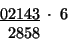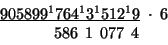# Speed Arithmetic - Multiplication by Six

## Introduction

Multiplication by 5, 6 and 7 uses a special concept of taking half'' of a digit. What this really means is taking half of an even number:or half of an odd number minus one - a better way of thinking about this is to just throw away the fractional leftover:It's a bit awkward to quote the word half'' here, but the concept is simple and this process can be practiced until it is done automatically and rapidly. Parents should clearly distinguish for children that the half'' discussed here is a special half'', not quite the same as the ordinary half (without quotes). Kids will get it, as they are used to the idea of let's pretend''. The above list of halves'' is complete, so committing this to memory is only a matter of practice.

## Rule for multiplying by six

Now that we have the concept of half'' down, we can go right to the rule for multiplying by six. The rule is:

Add to the number half'' the neighbor. Add 5 if the number is odd.

## Example with small even numbers

Let's take a simple example using all even numbers. Multiply 242 times 6:The digit 2 at the far right has no neighbor (so the neighbor is zero), so we just write it down:The next number is 4, and its neighbor is 2, so half'' of 2 is 1, and the next digit is 5 = 4 + 1:The next number is 2, and its neighbor is 4, so half'' of 4 is 2, and the next digit is 4 = 2 + 2:Finally, the next number is 0, and its neighbor is 2, so half'' of 2 is 1, and the next digit is 1 = 0 + 1:Thus we get 1452 as our product, which is the correct answer (check it with a calculator or do the multiplication the long way).

## Example with small even and odd numbers

We can try an example using both even and odd numbers next. Multiply 2143 by 6:The digit 3 at the far right has no neighbor, but it is odd, so we just add a 5 (3 + 5 = 8):The next number is 4 (an even number), and its neighbor is 3, so half'' of 3 is 1, and the next digit is 5 = 4 + 1:The next number is 1 (an odd number, will have to add 5), and its neighbor is 4, so half'' of 4 is 2, and the next digit is 8 = 1 + 2 + 5:The next number is 2 (an even number), and its neighbor is 1, so half'' of 1 is 0, and the next digit is 2 = 2 + 0:Finally, the next number is 0 (an even number), and its neighbor is 2, so half'' of 2 is 1, and the next digit is 1 = 0 + 1:The product is 12858, again the correct answer.

## Example with carrying a one

The above two examples used small numbers so we could see the way to do the method by itself. In the case of larger digits, the only additional complication is that of carrying a one when needed. For example, multiply 678 by 6:The digit 7 at the far right has no neighbor, and it is odd, so the first digit is 2 (7 + 5 = 12). The 1 is carried:Note carefully the placement of the carried 1 - we put it in between the next two numbers. This helps remind us that the next calculation needs an extra 1 added to it.

The next number is 8 (an even number), and its neighbor is 7, so half'' of 7 is 3. The next digit to add to the answer is 2 (8 + 3 + 1 = 12). The 1 is again carried:The next number is 6 (an even number), and its neighbor is 8, so half'' of 8 is 4. The next digit to add to the answer is 1 (6 + 4 + 1 = 11). The other 1 is carried:Finally, the next number is 0 (even number), and its neighbor is 6, so half'' of 6 is 3. The next digit to add to the answer is 4 (0 + 3 + 1):Therefore the product is 4122, the correct answer.

## Example with large numbers

We are now in a position to try an example with big numbers. This will allow us to see the interplay of all the elements shown above, and give a feel for how much work is involved in calculating a large product. In fact, the amount of work is not much more than for the examples above, since each step is just more of the same type of calculations done before. Multiply 90589976435129 by 6:The digit 9 at the far right has no neighbor, and is odd (so we'll have to add a 5). The first digit is 4 since 9 + 5 = 14. The 1 is carried:Next, we have a 2 (even), and neighbor 9, with half'' of 9 is 4. The next digit to add to the answer is 7 = 2 + 4 + 1:The next number in turn is 1 (odd, add 5), and its neighbor 2; half'' of 2 is 1. The next digit to add is 7 = 1 + 1 + 5:Next number is a 5 (odd, add 5), and its neighbor is 1, so half'' of 1 is 0. The next digit to add to the answer is 0, since 5 + 0 + 5 = 10, and the 1 is carried:The next number is 3 (odd, add 5), and its neighbor is 5, so half'' of 5 is 2. The next digit to add to the answer is 1, since 11 = 3 + 2 + 5 + 1. We carry the 1:Next, we find a 4 (even); its neighbor is 3, and half'' of 3 is 1. The next digit to add to the answer is 6 = 4 + 1 + 1:Then there is 6 (even), and its neighbor 4, with half'' of 4 equal to 2. We add to the answer the next digit 8 = 6 + 2:The next number is 7 (odd, add 5), neighbor 6, half'' of 6 is 3. The next digit to add to the answer is 5 (15 = 7 + 3 + 5) and the 1 is carried:Next number in turn is 9 (odd, add 5), and its neighbor is 7, so half'' of 7 is 3. Thus, we've got (including the carried 1) 9 + 3 + 5 + 1 = 18, so the 8 is written down as the next digit in the answer, and the 1 is carried:Next, we have another 9 (odd, add 5), the neighbor is 9, so half'' of 9 is 4. Including the carried 1, we've got 9 + 4 + 5 + 1 = 19. We write down the 9 and carry the 1:The number next in line is 8 (even), with neighbor 9, half'' of which is again 4. Since we are carrying another 1 from the previous step, we get 8 + 4 + 1 = 13, so write down the 2 and carry the 1:Now we have a 5 as the next number, which is odd and requires we add a 5. The neighbor is 8, with half'' equal to 4, so we add 5 + 4 + 5 + 1 = 15, meaning that the 5 is written down and the 1 is carried:The next-to-last number is 0 (even) with neighbor 5. Half'' of 5 is 2, so including the carried 1 we find that 0 + 2 + 1 = 3. We can just write this down:The last number is 9 again (odd, add 5), neighbor 0 with half'' equal to 0. That gives 9 + 5 = 14; we write down the 4 and carry the 1, but notice that we add 0 to the front of the number to hold the place (in effect adding an extra digit to the multiplicand, the invisible'' 0):The last thing we do is take the 0 (even), add half'' of 9 (in other words, add 4), and also add the carried 1, for 5 = 0 + 4 + 1:The correct answer is 543539858610774. Most calculators cannot handle numbers this large, and so a hand calculation was necessary to check this result. It is interesting to note that a clear advantage of the Trachtenberg method is that it can be used to compute arbitrarily large numbers. We should point out that errors are more likely with larger calculations; several were made as the above example was being developed. The point was to show a big computation in detail. Readers would be well advised to practice with more modest calculations until their proficiency is well established.

Recommended Books

The original, 1960 book that first brought Dr. Trachtenburg's work to the world's attention.  Well written, brief, with plenty of examples.

An inexpensive Dover book that covers the same kind of topics. I haven't read this one (yet), but I have many other Dover titles, and am always happy with them.